N1H111SM's Miniverse

# Mutual Information Maximization - Experiments

2020/05/13 ShareMaterials

# MI Estimation

## Correlated gaussian variables

Let $(X, Y)^T$ be a zero-mean Gaussian random vector with covariance matrix given by

Then the theoratical MI of the correated Gaussian distribution is given by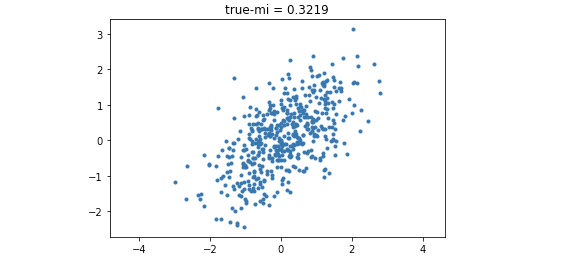## MI estimation with linear discriminator

We built a Linear-Discriminator-based MI estimator from scratch，其中采用的优化方法是梯度下降法。Linear discriminator会将两个sample $(a_n, b_n)$ 进行concatenation，通过一个简单的线性层即可就产生出scalar output. 因为是最简单的模型所以我们可以根据公式直接计算出每一步梯度的函数表示（具体看 KLD_estimate 和 gradient_descent 函数）。

## MI estimation with neural networks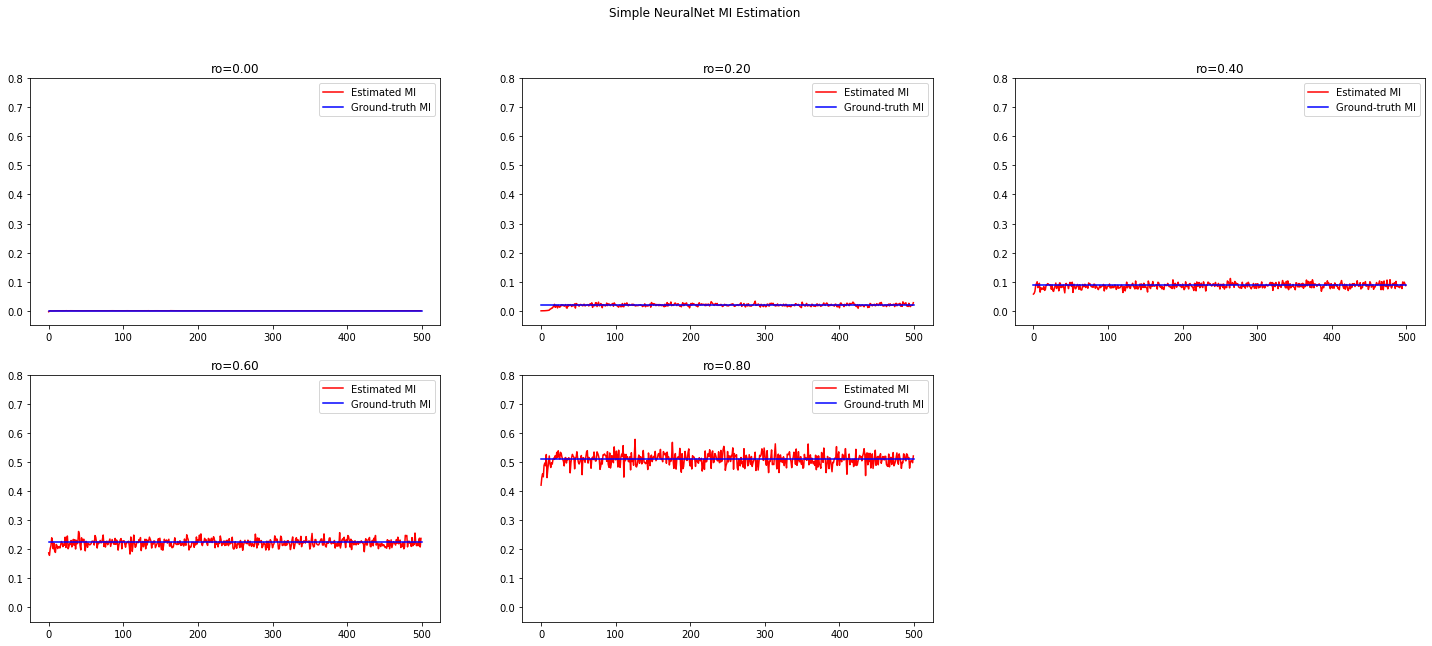# MI Maximization

## Inductive unsupervised representation learning via MI maximization

Assume we have the simulated unsupervised dataset $\tilde X = g(X)$, without knowing the form or any other knowledge of generation function $g$.

The target of the representation learning is to learn a model $M_{\psi}: \tilde X \rightarrow \hat X$ which maximize the mutual information between $\tilde X$ and $\hat X$. By doing so, we hope the model $M_\psi$ will help come into use one day in the future (using the knowledge of unlabeled data for coming supervised learning).

## Theoretical solution

Recall we use Donsker-Varadhan representation of KL divergence to estimate MI:

The goal of learning the best moddel $M$ becomes simultaneously estimating and maximizing MI.

where $\hat I$ is the total loss computed completely without supervision, i.e., given only $\tilde X$.

## Model implementation

Now we only need to treat them as a joint optimization problem. The internal logic of this unsupervised training setting is as follows:

• we have two smaller models - a inductive representation generator and an MI estimator - residing in the main model;
• when given a mini-batch of samples of $\tilde X$, the generator maps $\tilde X$ to $\hat X$;
• then the MI estimator treat the $(\tilde X, \hat X)$ as two distribution, estimate the mutual information of them, or more precisely, the lower bound of the their mutual information;
• when optimizing the MI estimation, the gradient of the objective also flows back to the generator;
• that’s why we say they are trained jointly.

## Simulating high-dimensional data

To simulate high-dimensional data, the generation function $g: x \rightarrow [f_1(x), f_2(x), \cdots, f_n(x)]$ is to generate high dimensional representation of $x$. For the sake of simplicity, the original $x$ is sampled from uniform distribution. And we can also add some noise into the model to make it more close to the real-world scenario.

One reason to play with such a toy setting is that now we can treat the problem of finding function $g^{-1}: [f_1(x), f_2(x), \cdots, f_n(x)] \rightarrow x$ as a supervised regression problem, and we have the ground-truth label of them!

The experimented data generation methods are as follows:

• $x \rightarrow [x^1-x^2, x^2-x^3, \cdots, x^k-x^{k+1}, \cdots, x^{n-1}-x^n, x^n]$, when reversed as a regression task, is linear-solvable.
• $x \rightarrow [x^1-x^2, x^2-x^3, \cdots, x^k-x^{k+1}, \cdots, x^{n-1}-x^n]$, when reversed as a regression task, is not linear-solvable, but can be easily approximated with linear model when $n$ is large.
• $x \rightarrow [\sin(x), \cos(x), \log(2+x), x^2, \sinh(x), \cosh(x), \tanh(x)]$, where each non-linear function $f$ can be further mapped to even more complex space by $J: f \rightarrow [f(x)^{\frac{1}{i+1}}|x: x \in \operatorname{dom}_f]$

## Sanity check

• Target: check whether the algorithm works.
• Method: using LR model to fit on the generated representation and the ground truth $(\tilde X, X)$, to see whether it’s MSE is minimized along the MI maximization.
• Data: We use $x$ -> $[x^1-x^2, x^2-x^3, \cdots, x^k-x^{k+1}, \cdots, x^{n-1}-x^n, x^n]$ to generate high-dimensional representation of $x$.

Data Generation:

The result of linear-solvable data $x \rightarrow [x^1-x^2, x^2-x^3, \cdots, x^k-x^{k+1}, \cdots, x^{n-1}-x^n, x^n]$ :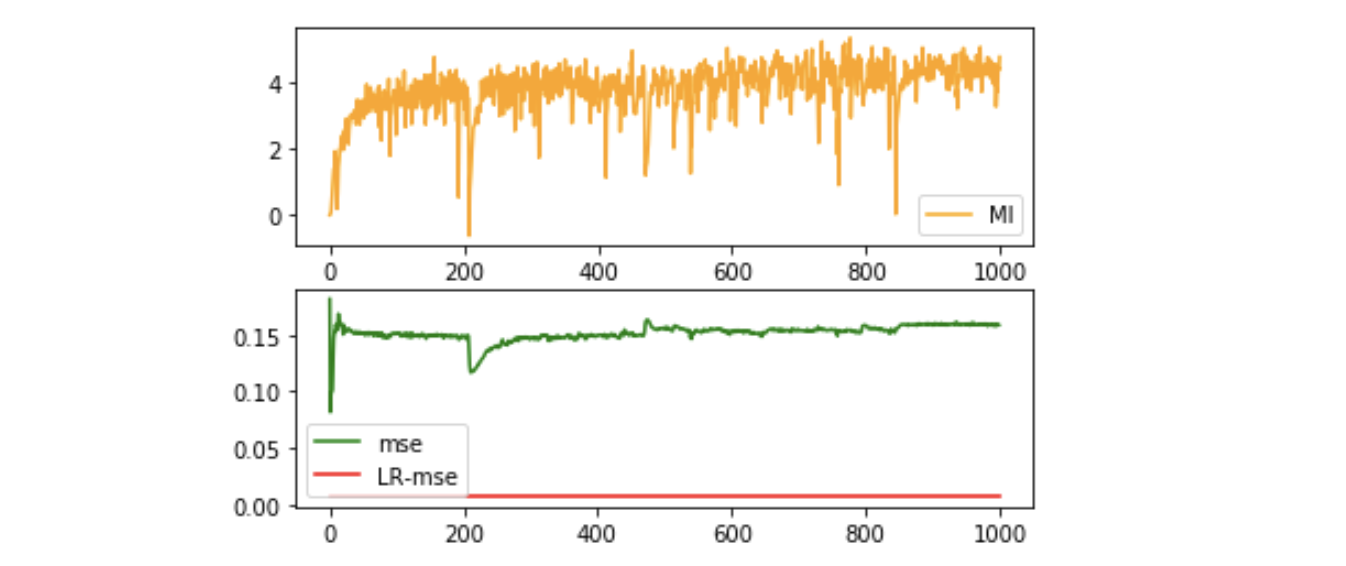## Influence of dimensionality of representation

• Target: find how the dimension of the representation matters.
• Method: use different output dimension.
• Data: We use $x$ -> $[x^1-x^2, x^2-x^3, \cdots, x^k-x^{k+1}, \cdots, x^{n-1}-x^n]$ to generate high-dimensional representation of $x$, which is not linear-solvable.

Data Generation:

Define train() function, train the model across different dimensionality setting:

Use the following code to visualize:

(1) Data without noise: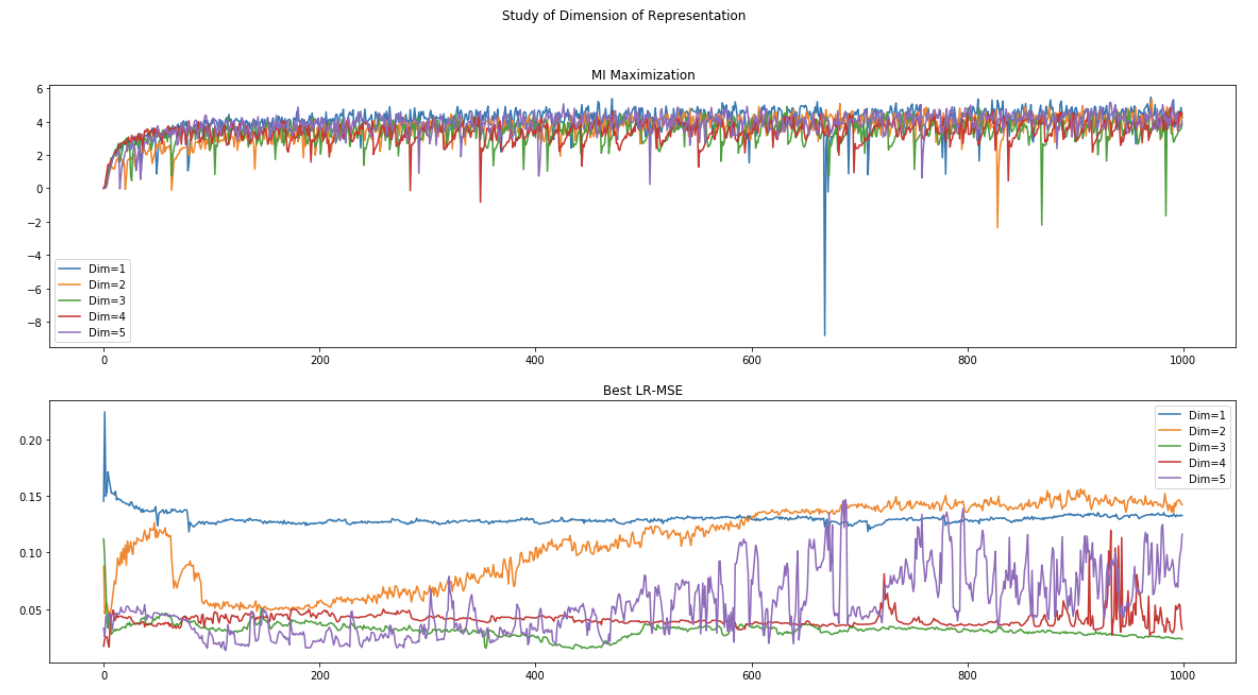(2) Data with noise (scale=0.05):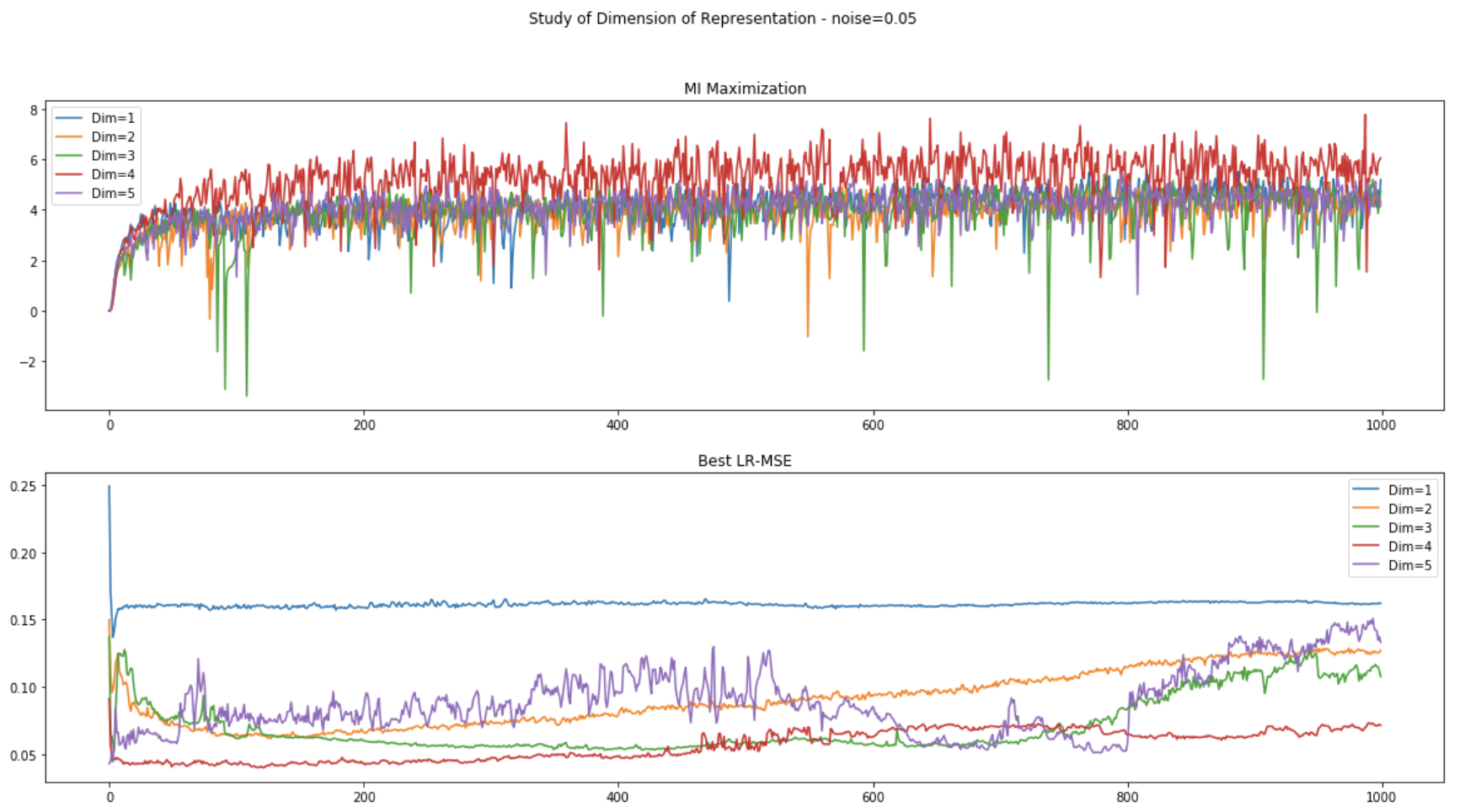## Unsupervised Representation Learning

• Target: Study the unsupervised learning using MI maximization.
• Method: unsupervised training dataset, supervised training dataset, testset.
• Data: We create the dataset (unsupervised/supervised/test dataset)

some tricks regarding generating a list of functions: 廖雪峰python教程

Data Generation given a list of functions:

We use the method introduced above to generate data of 20 dimension to evaluate the performance of the MI maximization method. Since the higher the dimension, the more linear-solvable the data is, we can expect that simple Linear Regression would solve it very well. Here is the result on different setting of noise.

### Results on linear-solvable data - study of noise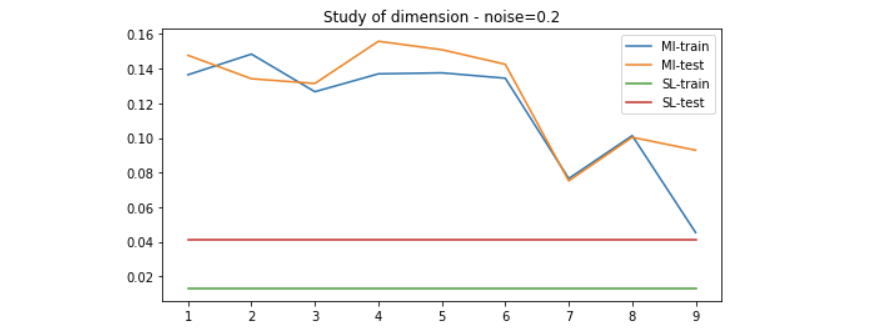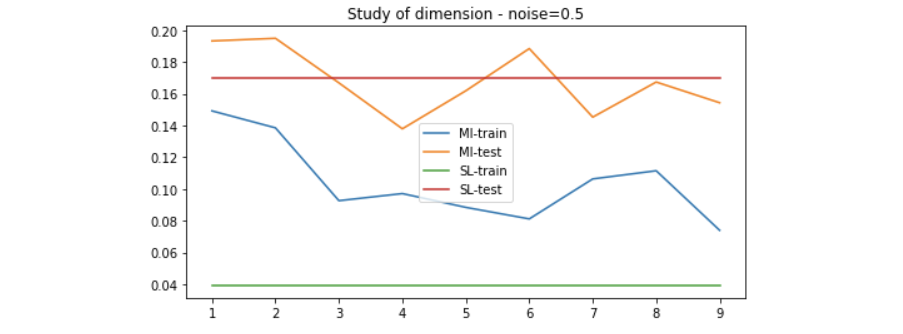### Results on non-linear-solvable data

Replace $\tilde X$ with non-linearity following: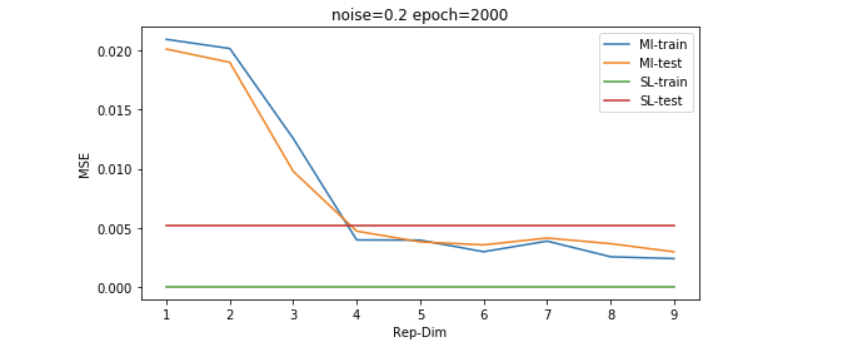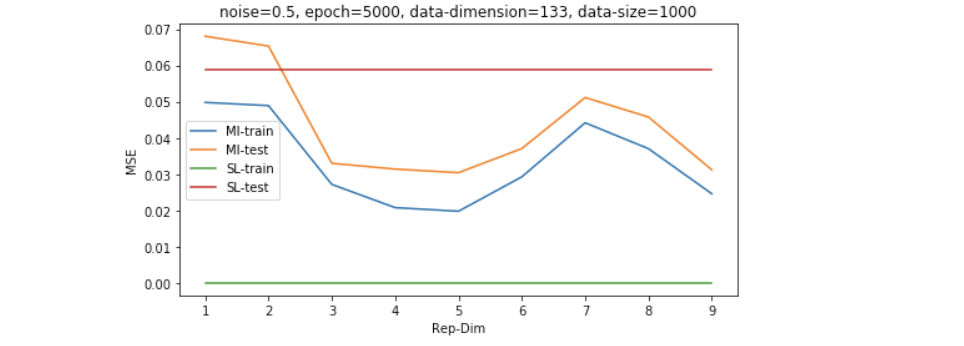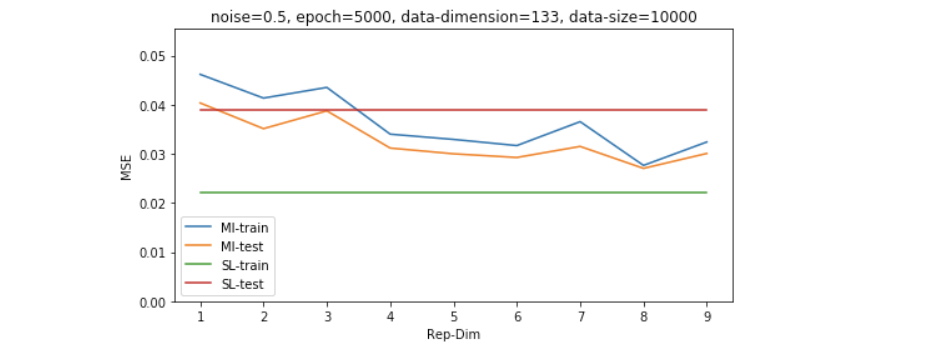### Results on shifted and scaled unsupervised data - study of dimensionality

Now all the data points are drawn from the exact same distribution, it’s time to check how the algorithm behave when there is shift/scale of the unsupervised data distribution.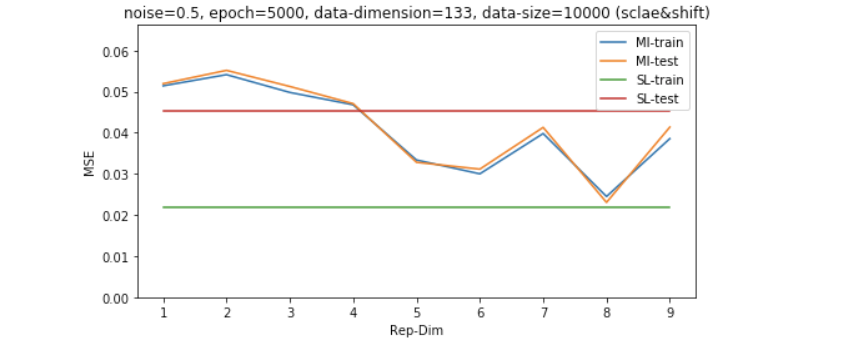## Analysis of the results

The result of which shows that the unsupervised learning via MI maximization is more stable across different data-noisy scearios including:

• small size of training data
• scale/shift in unsupervised data
• better performance with fewer parameters